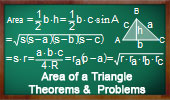# Plane Geometry: Area of a triangle, Theorems and Problems - Table of Content - Page 7Proposed Problem 110. Area of Contact Triangle.

The Pythagorean Curiosity Triangles and squares, fifteen conclusions.

Proposed Problem 103.Equilateral Triangle Area, Interior Point, Heron's Formula.

Proposed Problem 102.Equilateral Triangle Area, Interior Point.

Proposed Problem 97. Similar Triangles, Areas.

Proposed Problem 90. Quadrilateral and Triangle Areas, Midpoints.

Proposed Problem 89. Triangles of equal area, Midpoints.

Proposed Problem 88. Triangle and Quadrilateral Areas, Midpoints.

Proposed Problem 87. Area of Quadrilaterals and Midpoints of Diagonals. Area of triangles.

Proposed Problem 86. Intouch and Extouch Triangles, Areas.

Go to Page: Previous | 1 | 2 | 3 | 4 | 5 | 6 | 7 | 8 | Next